# RRB PO Quantitative Aptitude Quiz- 25

## RRB PO Prelims Quantitative Aptitude Quiz

Quantitative aptitude measures a candidate’s numerical proficiency and problem-solving abilities. It is the most important section of almost all competitive exams. Candidates are often stymied by the complexity of Quantitative Aptitude Questions but if they practice more and more questions it will become quite easy. So, here we are providing you with the RRB PO Quantitative Aptitude Quiz to enhance your preparation for your upcoming examination. Questions given in this RRB PO Quantitative Aptitude Quiz are based on the most recent and the latest exam pattern. A detailed explanation for each question will be given in this RRB PO Quantitative Aptitude Quiz. This RRB PO Quantitative Aptitude Quiz is entirely free of charge. This RRB PO Quantitative Aptitude Quiz will assist aspirants in achieving a good score in their upcoming examinations.

Directions (1-5): What should come in place of question mark (?) in the following questions?

1. 1528 + 525 ÷ 25 – 840 = 510 + ?

(a) 199

(b) 299

(c) 159

(d) 189

(e) 165

Ans. a

Exp.

1528 + 21 – 840 – 510 = ?

? = 1549 – 1350

? = 199(a) 259

(b) 169

(c) 157

(d) 129

(e) 141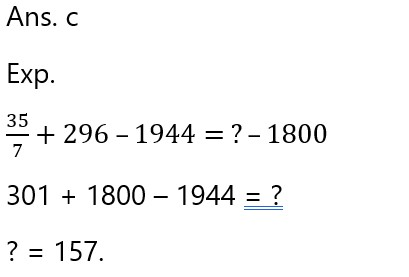1. 65% of 180 + ?% of 210 = 80% of 225

(a) 45

(b) 30

(c) 40

(d) 50

(e) 25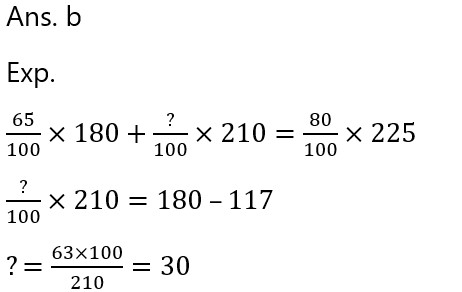(a) 145

(b) 115

(c) 120

(d) 135

(e) 125

Ans. e

Exp.

1500 + 140 – 1 + ? = 1764

? = 1764 – 1639

? = 125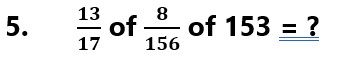(a) 8

(b) 12

(c) 7

(d) 6

(e) 41. The simple interest on a certain sum for 2 years at 8% per annum is Rs. 225 less than the compound interest on the same sum for 2 years at 10% per annum. The sum is:

(a) Rs. 3200

(b) Rs. 4200

(c) Rs. 4000

(d) Rs. 3600

(e) Rs. 4500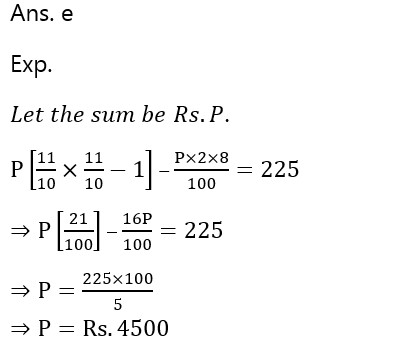7.       A, B and C completed a work costing Rs. 2400. A, B and C can complete the work alone in 12, 20 and 30 days respectively. If A, B and C together finish the work then how much amount will be received by B per day?

(a) Rs. 210

(b) Rs. 180

(c) Rs. 90

(d) Rs. 120

(e) Rs. 1501. If simple interest on a certain sum of money for three years is Rs. 450 and the compound interest on the same sum at the same rate for 2 years is Rs. 309, then the principal invested in rupees is :

(a) Rs. 3000

(b) Rs. 1875

(c) Rs. 1500

(d) Rs. 2250

(e) Rs. 2500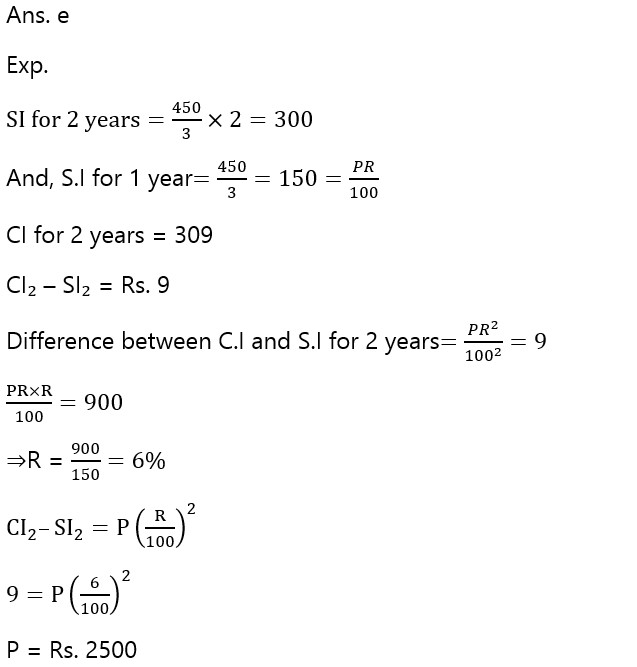1. Abhijeet sell a mobile to Ranveer at 20% loss. Next day Abhijeet change his mind and purchase the same mobile from Ranveer. But Ranveer sell it at 20% profit. If actual cost price of mobile is 12000 then find the total actual amount paid by Abhijeet.

(a) 23520

(b) 13920

(c) 11520

(d) 9600

(e) 48001. A train met with an accident 120 km from station A. It completed the remaining journey at 5/6 of its previous speed and reached 2 hours late at station B. Had the accident taken place 300 km further, it would have been only 1 hour late, what is the speed of the train?

(a) 50 kmph

(b) 60 kmph

(c) 45 kmph

(d) 58 kmph

(e) 65 kmph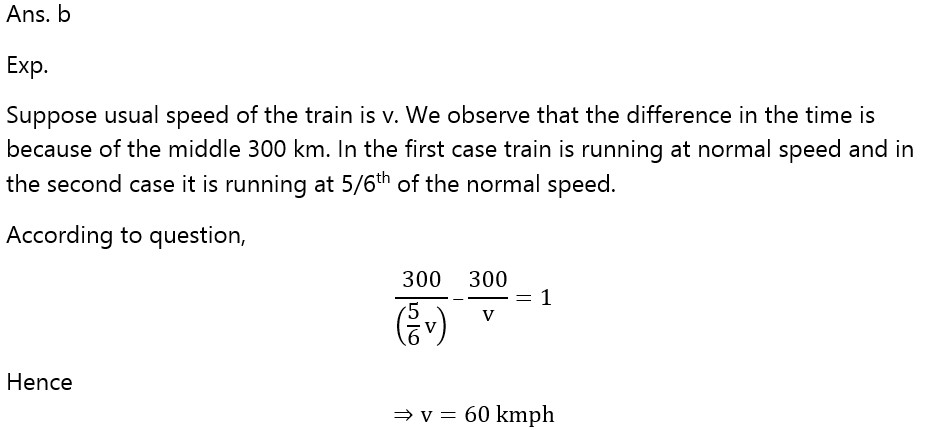###Click to Buy Bank MahaCombo PackageRecommended PDF’s for:

#### Most important PDF’s for Bank, SSC, Railway and Other Government Exam : Download PDF Now

AATMA-NIRBHAR Series- Static GK/Awareness Practice Ebook PDF Get PDF here
The Banking Awareness 500 MCQs E-book| Bilingual (Hindi + English) Get PDF here
AATMA-NIRBHAR Series- Banking Awareness Practice Ebook PDF Get PDF here
Computer Awareness Capsule 2.O Get PDF here
AATMA-NIRBHAR Series Quantitative Aptitude Topic-Wise PDF Get PDF here
AATMA-NIRBHAR Series Reasoning Topic-Wise PDF Get PDF Here
Memory Based Puzzle E-book | 2016-19 Exams Covered Get PDF here
Caselet Data Interpretation 200 Questions Get PDF here
Puzzle & Seating Arrangement E-Book for BANK PO MAINS (Vol-1) Get PDF here
3